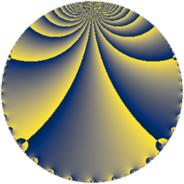# Properties

 Label 784.4.pLevel $784$ Weight $4$ Character orbit 784.p Rep. character $\chi_{784}(31,\cdot)$ Character field $\Q(\zeta_{6})$ Dimension $120$ Sturm bound $448$

# Related objects

## Defining parameters

 Level: $$N$$ $$=$$ $$784 = 2^{4} \cdot 7^{2}$$ Weight: $$k$$ $$=$$ $$4$$ Character orbit: $$[\chi]$$ $$=$$ 784.p (of order $$6$$ and degree $$2$$) Character conductor: $$\operatorname{cond}(\chi)$$ $$=$$ $$28$$ Character field: $$\Q(\zeta_{6})$$ Sturm bound: $$448$$

## Dimensions

The following table gives the dimensions of various subspaces of $$M_{4}(784, [\chi])$$.

Total New Old
Modular forms 720 120 600
Cusp forms 624 120 504
Eisenstein series 96 0 96

## Trace form

 $$120q - 540q^{9} + O(q^{10})$$ $$120q - 540q^{9} + 1248q^{25} + 168q^{29} + 108q^{33} + 252q^{37} - 1980q^{45} + 588q^{53} + 5256q^{57} + 900q^{61} - 1812q^{65} + 324q^{73} - 6696q^{81} - 8952q^{85} - 1908q^{89} + 1980q^{93} + O(q^{100})$$

## Decomposition of $$S_{4}^{\mathrm{new}}(784, [\chi])$$ into newform subspaces

The newforms in this space have not yet been added to the LMFDB.

## Decomposition of $$S_{4}^{\mathrm{old}}(784, [\chi])$$ into lower level spaces

$$S_{4}^{\mathrm{old}}(784, [\chi]) \cong$$ $$S_{4}^{\mathrm{new}}(28, [\chi])$$$$^{\oplus 6}$$$$\oplus$$$$S_{4}^{\mathrm{new}}(112, [\chi])$$$$^{\oplus 2}$$$$\oplus$$$$S_{4}^{\mathrm{new}}(196, [\chi])$$$$^{\oplus 3}$$